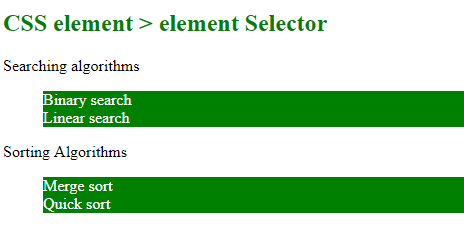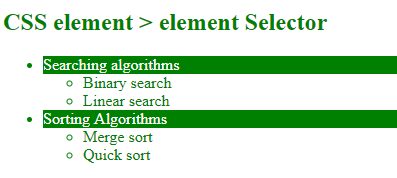Open In App

# What is greater-than sign (>) selector in CSS?

The greater than sign (>) selector in CSS is used to select the element with a specific parent. It is called as element > element selector. It is also known as the child combinator selector which means that it selects only those elements which are direct children of a parent. It looks only one level down the markup structure and not further deep down. Elements which are not the direct child of the specified parent is not selected.

Syntax:

```element > element {
// CSS Property
}```

Example-1: This example describes the greater than > selector.

 ` ``<``html``> ``    ``<``head``> ``        ``<``title``> ``            ``CSS element > element Selector ``        `` ``        ``<``style``> ``            ``ul > li { ``                ``color:white; ``                ``background: green; ``            ``} ``        `` ``    `` ``    ``<``body``> ``        ``<``h2` `style` `= ``"color:green;"``> ``            ``CSS element > element Selector ``        `` ``         ` `        ``<``div``>Searching algorithms ``         ` `        ``<``ul``> ``            ``<``li``>Binary search ``            ``<``li``>Linear search ``        `` ``         ` `        ``<``p``>Sorting Algorithms ``        ``<``ul``> ``            ``<``li``>Merge sort ``            ``<``li``>Quick sort ``        `` ``    `` `` `

Output:Example 2: This example describes the greater than > selector.

 ` ``<``html``> ``    ``<``head``> ``        ``<``title``> ``            ``CSS element > element Selector ``        `` ``         ` `        ````        ``<``style``> ``            ``li > div { ``                ``color:white; ``                ``background: green; ``            ``} ``            ``ul > li {``                ``color: green;``            ``}``        `` ``    `` ``     ` `    ``<``body``> ``        ``<``h2` `style` `= ``"color:green;"``> ``            ``CSS element > element Selector ``        `` ``         ` `        ``<``ul``>``            ``<``li``>``                ``<``div``>Searching algorithms ``         ` `                ``<``ul``> ``                    ``<``li``>Binary search ``                    ``<``li``>Linear search ``                ````            `` ``         ` `            ``<``li``>``                ``<``div``>Sorting Algorithms ``                ``<``ul``> ``                    ``<``li``>Merge sort ``                    ``<``li``>Quick sort ``                ````            ````        ````    `` `` `

Output:CSS is the foundation of webpages, is used for webpage development by styling websites and web apps.You can learn CSS from the ground up by following this CSS Tutorial and CSS Examples.